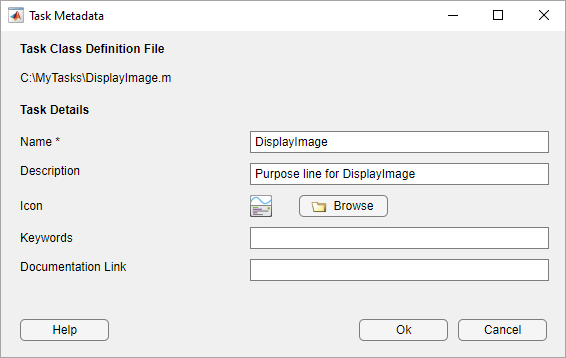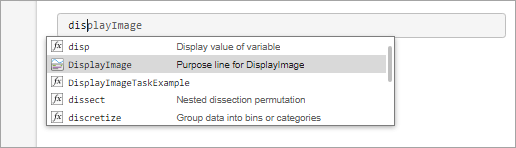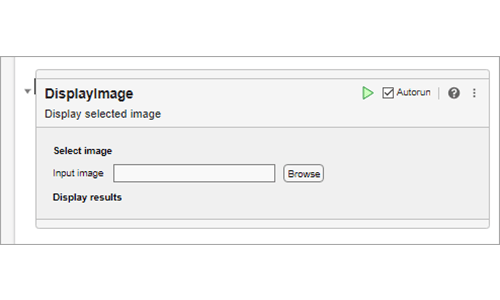# generateCode

Generate code for instance of Live Editor task subclass

## Syntax

```[code,taskoutputs] = generateCode(obj) ```

## Description

`[code,taskoutputs] = generateCode(obj)` generates the MATLAB® commands and output for the task. This method executes when the state of the task changes. The generated code displays in the code section of the task. When the live script section containing the task runs, the Live Editor uses the generated code to run the task. Define this method in a method block with no arguments, along with the `get.Summary`, `get.State`, `set.State`, and `reset` methods.

## Input Arguments

expand all

Object of the class that inherits from the `matlab.task.LiveTask` base class.

## Output Arguments

expand all

Generated code for the task, returned as a string array or character array.

Output variables generated by the task, returned as a cell array. If the task does not generate output, return `taskoutputs` as an empty cell array.

## Attributes

 `Abstract` `true`

To learn about attributes of methods, see Method Attributes.

## Examples

expand all

Define a class called `DisplayImage` that creates a custom Live Editor task for displaying an image.

To define the class, create a file called `DisplayImage.m` that contains the following class definition with these features:

• `State` and `Summary` public properties that store the current state of the task and a dynamic summary of what the task does.

• Private properties that store the edit field and button for selecting an image.

• A `setup` method that initializes the task.

• A `generateCode` method that updates the generated code for the task when the selected image changes.

• `get.Summary`, `get.State`, and `set.State` methods for getting and setting the summary and state of the task.

• A `getImageFile` method that prompts the user to select an image to display.

• A `reset` method that resets the state of the task.

```classdef DisplayImage < matlab.task.LiveTask properties(Access = private,Transient) FileNameEditField matlab.ui.control.EditField BrowseButton matlab.ui.control.Button end properties(Dependent) State Summary end methods(Access = protected) function setup(task) createComponents(task); setComponentsToDefault(task); end end methods function [code,outputs] = generateCode(task) if isempty(task.FileNameEditField.Value) % Not have enough information to generate code, % return empty values code = ""; outputs = {}; return end outputs = {"im"}; code = "% Get Image"; code = code + newline + outputs{1} + " = imread('" ... + task.FileNameEditField.Value + "');"; code = code + newline + newline + "% Visualize results" + ... newline + "figure" + newline; code = code + "imshow(" + outputs{1} + ");"; end function summary = get.Summary(task) if isempty(task.FileNameEditField.Value) summary = "Display selected image"; else [~,name,~] = fileparts(task.FileNameEditField.Value); summary = "Display image '" + name + "'"; end end function state = get.State(task) state = struct; state.FileNameEditFieldValue = task.FileNameEditField.Value; end function set.State(task,state) task.FileNameEditField.Value = state.FileNameEditFieldValue; inputImageFile(task); end function reset(task) setComponentsToDefault(task); inputImageFile(task); end end methods(Access = private) function createComponents(task) task.LayoutManager.RowHeight = ["fit" "fit" "fit" "fit"]; task.LayoutManager.ColumnWidth = "fit"; % Row 1: Select image section label uilabel(g,"Text","Select image","FontWeight","bold"); % Row 2: Select data section components inputgrid = uigridlayout(g,"RowHeight","fit","ColumnWidth", ... {"fit",200,"fit"},"Padding",0); uilabel(inputgrid,"Text","Input image"); task.FileNameEditField = uieditfield("Parent",inputgrid, ... "Editable",false); task.BrowseButton = uibutton("Parent",inputgrid,"Text","Browse", ... "ButtonPushedFcn",@task.inputImageFile); % Row 3: Display results section label uilabel(g,"Text","Display results","FontWeight","bold"); end function setComponentsToDefault(task) task.FileNameEditField.Value = ""; end function inputImageFile(task,~,~) % Display uigetfile dialog box filterspec = ["*.jpg;*.tif;*.png;*.gif","All Image Files"]; [f,p] = uigetfile(filterspec); % Make sure user did not cancel uigetfile dialog box if (ischar(p)) fileName = [p f]; task.FileNameEditField.Value = fileName; end notify(task,"StateChanged"); end end end ```

Next, configure the task metadata by calling the `matlab.task.configureMetadata` function and selecting the `DisplayImage.m` file. The Task Metadata dialog box opens with all of the required task metadata details prepopulated.Select OK to use the prepopulated metadata details. MATLAB creates a folder named `resources` inside the folder containing your task class definition file. Inside the `resources` folder, MATLAB generates a file named `liveTasks.json`. Add the folder containing the task class definition file to the MATLAB path by calling the `addpath` function or using the Add Folder button in the Set Path dialog box. To make your task available in the Live Editor in future MATLAB sessions, save the path by calling the `savepath` function or using the Save button in the Set Path dialog box.

Add the task to a live script. On a code line, type `display`. MATLAB shows a list of suggested matches.Select Display Image from the list. MATLAB adds the Display Image task to the live script.## Version History

Introduced in R2022a